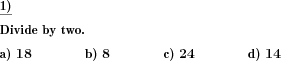Custom math worksheets at your fingertips# Details for problem "Halve numbers"

Quickname: 1718

Elementary School, Primary School, Junior High School, Middle School.

## Summary

Divide numbers by two, whole numbers or with decimal places.

## Example## Description

This is a division by two problem.

A series of numbers is shown, these have to be divided by two. The number range can be chosen, and the number of decimals, if desired.

There will be no remainders left, and the quotient will have no more decimals that the dividend.

The number of problems can be specified.

Download free printable worksheets for this math problem here. The worksheet contains the problems only, the solution sheet includes the answers. Just click on the respective link.

•Worksheet 1Solution sheet with answers
•Worksheet 2Solution sheet with answers
•Worksheet 3Solution sheet with answers

If you can not see the solution sheets for download, they may be filtered out by an ad blocker that you may have installed. If this is the case, please allow ads for this page and reload the page. The solution sheets will then reappear.

• Do these sample worksheets do not really fit?
• Do you need more math worksheets, with a different level of difficulty?
• Would you like to combine different problems on a worksheet and adjust them to your needs?
• As a teacher, you can put together your own worksheets using the automatically generated math problems provided.
With a free initial credit, you can start creating your own math worksheets in a few minutes.

You can try it for free! Register here, to create custom worksheets now!

## Customization options for this problem

Parameter
Possible values
Number of problems
1, 2, 3, 4, 5, 6, 7, 8, 9, 10
Number range
Numeric value from 1 to 999999.
Decimal places
0, 1, 2, 3

## Similar problems

Remark
Description
General division case
A decimal fraction has to be divided by another decimal fraction.
General division case for whole numbers
Division of two whole numbers, with remainder, written long division method illustrated.